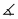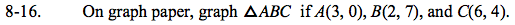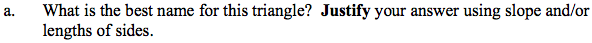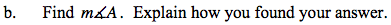Home > GC > Chapter 8 > Lesson 8.1.2 > Problem8-16

8-16.
1. On graph paper, graph ΔABC if A(3, 0), B(2, 7), and C(6, 4). 8-16 HW eTool (Desmos). Homework Help ✎

1. What is the best name for this triangle? Justify your answer using slope and/or lengths of sides.

2. Find mA. Explain how you found your answer.What are the characteristics of the triangle? What kind of triangle has these?

$\text{Remember slope is }\frac{\Delta y}{\Delta x}\text{, and for length use the Pythagorean Theorem.}$

This is an isosceles right triangle because 2 side lengths are equal and 2 sides a perpendicular.What trigonometric ratio can you use to find the measure of the angle?
Refer to the Math Notes Box in Lesson 5.1.2 for the trigonometry ratios and don't forget about inverse trigonometry.

Use the eTool below to visualize the problem and answer questions (a) and (b).
Click the link at right for the full version of the eTool: GC 8-16 HW eTool